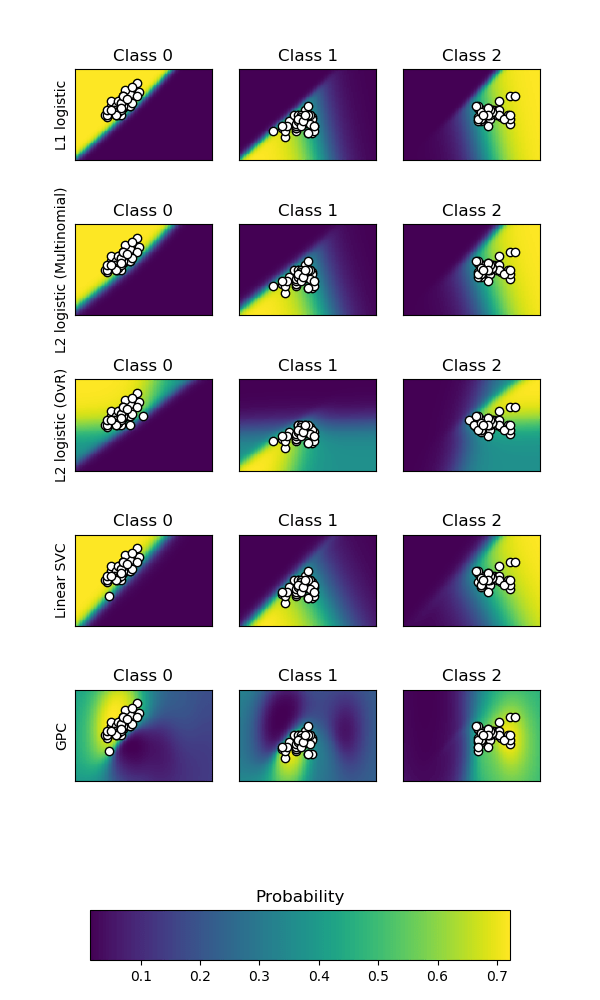/scikit-learn

# Plot classification probability

Plot the classification probability for different classifiers. We use a 3 class dataset, and we classify it with a Support Vector classifier, L1 and L2 penalized logistic regression with either a One-Vs-Rest or multinomial setting, and Gaussian process classification.

Linear SVC is not a probabilistic classifier by default but it has a built-in calibration option enabled in this example (`probability=True`).

The logistic regression with One-Vs-Rest is not a multiclass classifier out of the box. As a result it has more trouble in separating class 2 and 3 than the other estimators.Out:

```Accuracy (train) for L1 logistic: 82.7%
Accuracy (train) for L2 logistic (Multinomial): 82.7%
Accuracy (train) for L2 logistic (OvR): 79.3%
Accuracy (train) for Linear SVC: 82.0%
Accuracy (train) for GPC: 82.7%
```
```print(__doc__)

# Author: Alexandre Gramfort <alexandre.gramfort@inria.fr>

import matplotlib.pyplot as plt
import numpy as np

from sklearn.metrics import accuracy_score
from sklearn.linear_model import LogisticRegression
from sklearn.svm import SVC
from sklearn.gaussian_process import GaussianProcessClassifier
from sklearn.gaussian_process.kernels import RBF
from sklearn import datasets

X = iris.data[:, 0:2]  # we only take the first two features for visualization
y = iris.target

n_features = X.shape

C = 10
kernel = 1.0 * RBF([1.0, 1.0])  # for GPC

# Create different classifiers.
classifiers = {
'L1 logistic': LogisticRegression(C=C, penalty='l1',
solver='saga',
multi_class='multinomial',
max_iter=10000),
'L2 logistic (Multinomial)': LogisticRegression(C=C, penalty='l2',
solver='saga',
multi_class='multinomial',
max_iter=10000),
'L2 logistic (OvR)': LogisticRegression(C=C, penalty='l2',
solver='saga',
multi_class='ovr',
max_iter=10000),
'Linear SVC': SVC(kernel='linear', C=C, probability=True,
random_state=0),
'GPC': GaussianProcessClassifier(kernel)
}

n_classifiers = len(classifiers)

plt.figure(figsize=(3 * 2, n_classifiers * 2))

xx = np.linspace(3, 9, 100)
yy = np.linspace(1, 5, 100).T
xx, yy = np.meshgrid(xx, yy)
Xfull = np.c_[xx.ravel(), yy.ravel()]

for index, (name, classifier) in enumerate(classifiers.items()):
classifier.fit(X, y)

y_pred = classifier.predict(X)
accuracy = accuracy_score(y, y_pred)
print("Accuracy (train) for %s: %0.1f%% " % (name, accuracy * 100))

# View probabilities:
probas = classifier.predict_proba(Xfull)
n_classes = np.unique(y_pred).size
for k in range(n_classes):
plt.subplot(n_classifiers, n_classes, index * n_classes + k + 1)
plt.title("Class %d" % k)
if k == 0:
plt.ylabel(name)
imshow_handle = plt.imshow(probas[:, k].reshape((100, 100)),
extent=(3, 9, 1, 5), origin='lower')
plt.xticks(())
plt.yticks(())
idx = (y_pred == k)
if idx.any():
plt.scatter(X[idx, 0], X[idx, 1], marker='o', c='w', edgecolor='k')

ax = plt.axes([0.15, 0.04, 0.7, 0.05])
plt.title("Probability")
plt.colorbar(imshow_handle, cax=ax, orientation='horizontal')

plt.show()
```

Total running time of the script: ( 0 minutes 12.541 seconds)

Gallery generated by Sphinx-Gallery